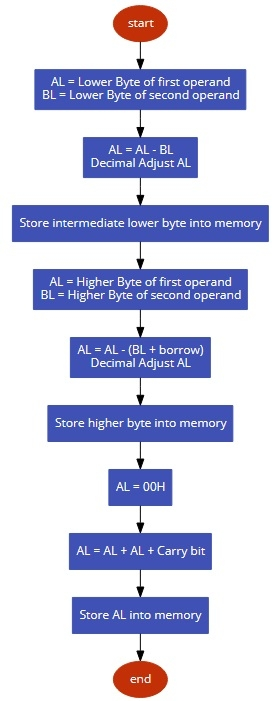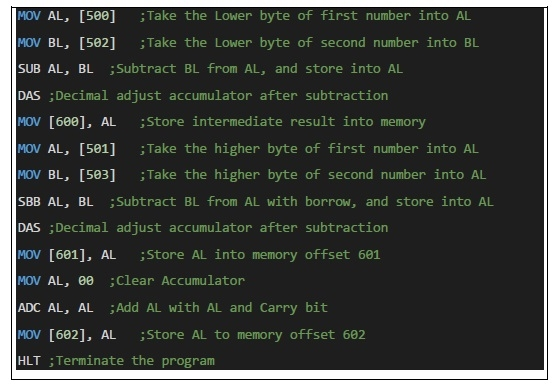# 8086 program to subtract two 16 bit BCD numbers

MicroprocessorMicrocontroller8086

In this program we will see how to subtract two 16-bit BCD numbers.

## Problem Statement

Write 8086 Assembly language program to subtract two 16-bit BCD numbers stored in memory offset 500H – 501H and 502H – 503H.

## Discussion

Here we are adding the 16-bit data byte by byte. At first we are subtracting lower byte and perform the DAS instruction, then Subtract higher bytes with borrow, and again DAS to adjust. The final result is stored at location offset 600H, and if borrow is present, it will be stored at 601H.

We are taking two numbers 8523 - 7496 = 1027

Data

500
23
501
85
502
96
503
74

## Flow Diagram## Program